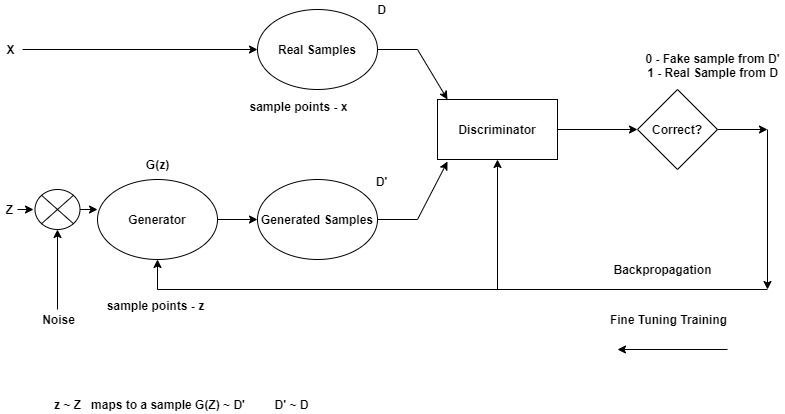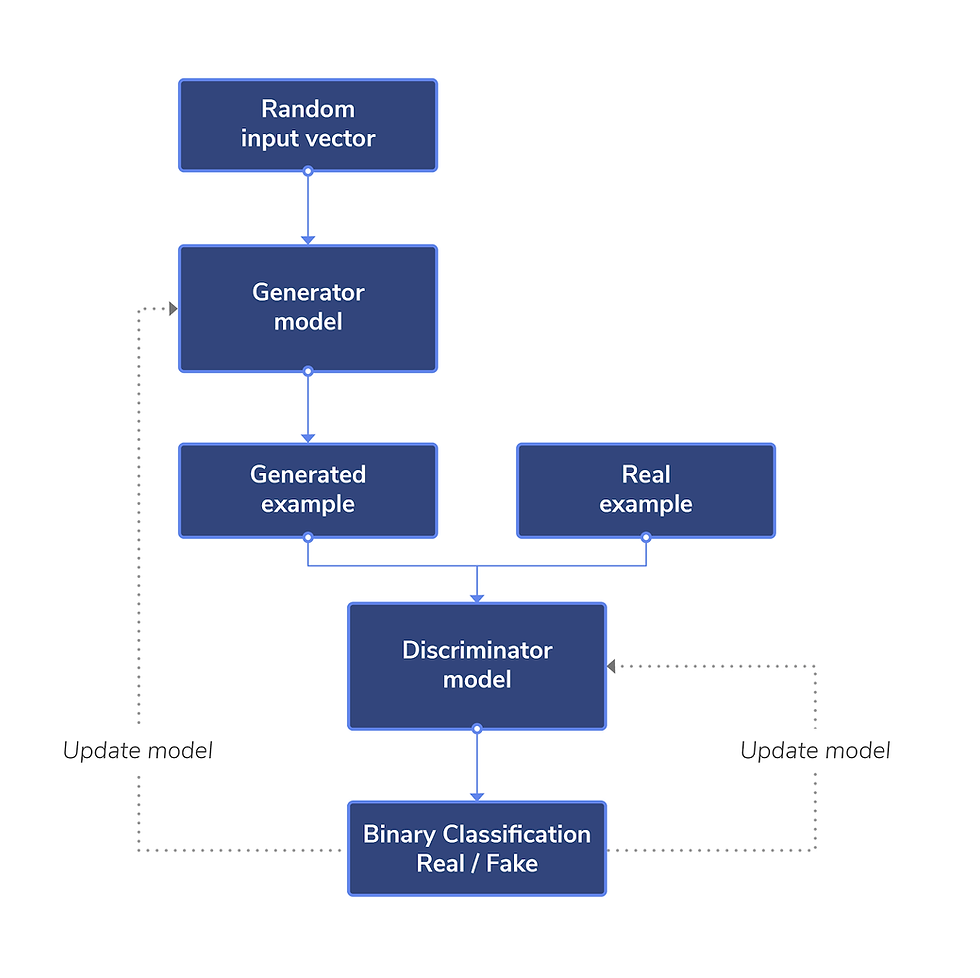top of page
Search
• Anisha Mohanty

# GANs - the light switch of AI, we have been searching for…A Generative Adversarial Network, or GAN, is a form of generative modelling neural network architecture.

Using a model to produce new examples that are plausible to come from an established distribution of samples, such as generating new images that are identical but distinct from a dataset of existing photographs, is referred to as generative modelling.

A GAN is a generative model that uses two neural network models to train it. The "generator" or "generative network" model, for example, learns to produce new plausible samples. The "discriminator" or "discriminative network" model, on the other hand, learns to distinguish generated examples from real examples.

The two models are set up in a contest or game (in the context of game theory) in which the generator model attempts to deceive the discriminator model, and the discriminator is given both real and generated sample examples.

The generative model can then be used to produce new plausible samples on demand after it has been educated.

In short GANs are basically deep neural network architectures comprised of two neural networks fighting against each other and generating data, mimicking some probability distribution functions.

The term generative in GANs is because these networks generate some probability distribution function that is close to the original data. The term Adversarial means conflict/opposition in English dictionary. It justifies its meaning in GANs because here there are two networks discriminator and generator that fight with earth other to learn the distribution function.

Discriminative Model : It discriminates between two different classes of data (output - 0/1 for fake and real respectively).

Generative Model (G) : This neural network model is trained on X sample data with x sample points from true distribution D, gives random distribution Z with sample points z, that produces a new distribution D’. D’ produced from the generative samples is close to the original distribution D from the original sample data.

How do GANs really work?X - original sample data

x - sample points in X data set

Z - artificial data created by using random distribution to generate samples and distribution close to the original distribution D

D - original unknown Probability distribution function to create a boundary surrounding x data points

D’ - Generated distribution for the new sample data points z(of Z)

G(z) - Generator generated samples

Objective of the Discriminator is to distinguish real instances from fake ones and produce output as 1 for real instances and 0 for fake instances.

But the objective of the Generator module is to confuse the discriminator module from distinguishing between the fake and real samples by adjusting its weights and bias.

How are GANs trained?

For the real instances and the artificial instances, we must get the output as 1 and 0 respectively.

Learning Mechanism :

Training the discriminator -

1. Feed noise to the Generator

2. Generate Artificial instances

3. Label them as y = 0

4. Take real instances and label them as y = 1

5. Feed both to the discriminator and allow the discriminator model to distinguish between the two.

Training the Generator -

1. Fix Discriminator weights and biases

2. Again create z sample points from Z data using random distribution

3. Feed this to the Generator

4. Create artificial instances and label them y = 1

5. This is to fool the discriminator to produce output as 1

6. If this step fails, then update and adjust the weights and biases of the generator model (Backpropagation).

Loss Function Calculation -

1. If the discriminator fails to distinguish the real and fake instances, then an error is generated

2. For that error calculate the loss function and error is backpropagated

3. Generative model weights are adjusted because generator data points couldn't fool the discriminator. (Our objective is to train the discriminator to distinguish the real and artificial outputs by the labels of 1 and 0, but at a certain point of time and after many iterations and weight adjustments, the generator must produce data points that are somehow similar to the original sample data points. Hence we have to adjust the weights of the generator until we get the discriminator output to be 0.5, which represents that we have successfully fooled the Discriminator model)Loss Function Derivation

From Binary Cross Entropy,

`L(ŷ, y) =[y log ŷ + (1 - y) log (1 - ŷ)]`

Where, y - original sample point

ŷ - reconstructed data point

For data coming from the discriminator,

Label pdata(x) as y = 1 and pg(z) as ŷ = D(x)

Hence,

`L(D(x), 1) = log(D(x))   ……….(A)`

For data coming from generator,

Label pdata(x) as y = 0 and pg(z) as ŷ = D(G(z))

Hence,

``` L(D(G(z)), 0) = (1 - 0) log (1 - D(G(z)))
= log (1 - D(G(z))) ………..(B)```

In order to classify fake and real outputs correctly by the help of the discriminator, maximize (A) and (B)…Graph of log(D(x)) - here the maximum value can be D(x) = 1Graph of log(1 - D(G(z))) - here the

maximum value can be D(G(z)) = 0

`D = max{log (D(x)) + log (1 - D(G(z)))}`

In order to fool the discriminator, (A) and (B) must be minimized…

1. Doesn't depend on D(G(z))

2. log (1 - D(G(z))) is minimized

`G = min{log (D(x)) + log (1 - D(G(z)))} `

Hence the overall loss function for one instance can be written as…

``` min max {log (D(x)) + log (1 - D(G(z)))} -----> for one instance
G   D ```

For calculating the loss function of all the instances or data points, we have to take the Expectation value,

``` min max V(D, G) = min max {E[log (D(x))] + E[log (1 - D(G(x)))]}
G   D             G   D```

GANs, the real Breakthrough in AI

GANs have made a lot of noise in the science world. Many comparisons can be made between GANs and previous networks that have been robust enough to survive competition while still being versatile enough to integrate with new methodologies. These networks allow for unanticipated hybridization, and the simplicity with which they combine pre-existing models makes them efficient and unmistakable.

GANs can also learn to synthesize speech based on complex human gestures, head posture, and eye gaze. This can also be used to detect depression or other mental illnesses in their early stages. GANs' meteoric rise in popularity has been followed by an equivalent increase in their ability to penetrate domains previously untouched by AI.

GANs can do it all, from developing super-realistic expressions to diving deep into deep space, from bridging the human-machine empathy gap to incorporating new art forms. If AI research is akin to probing a dark space, GANs could be the light switch we've been searching for.

What we learned…

• This was a brief overview which covers the theoretical aspects and mathematics behind Generative Adversarial Networks.

• Before diving deep into the code and implementation part we must be familiar with the concepts that hold the code’s barebone structure.